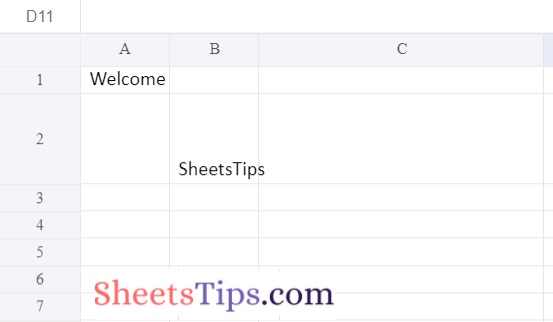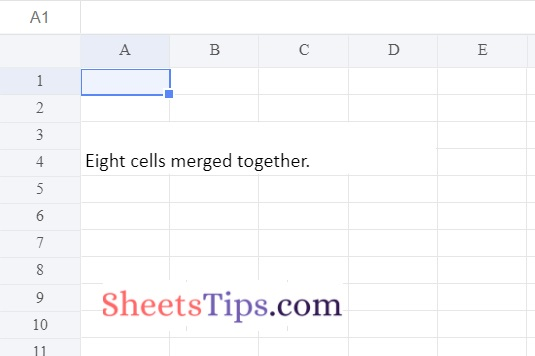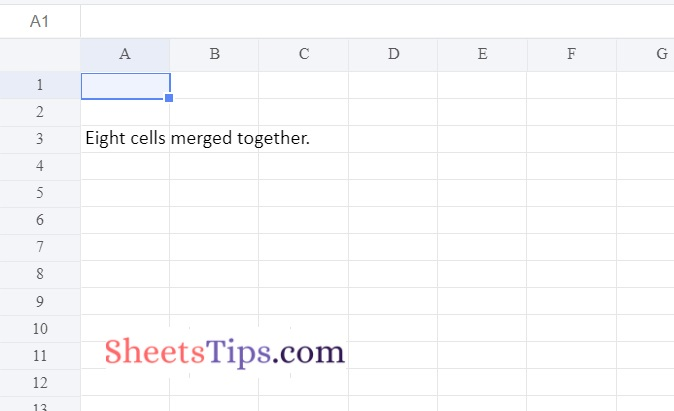# How to Adjust Rows and Columns of an Excel File using openpyxl Module in Python?

Python is a powerful programmable language that allows us to perform various operations on Excel, from simple to complicated. Adjusting rows and columns in an Excel file just takes seconds. But we can also adjust the rows and columns in Excel just by using the openpyxl module in Python. Surprised? Well, the detailed steps and examples on how to adjust the column and row width in Excel are provided on this page. However, before going through the tutorial, let us understand the components of worksheet objects.

Row dimension and column dimension, properties that regulate row heights and column widths, are present in worksheet objects. The values in a dictionary are comparable to the row and column dimensions of a sheet. RowDimension objects are contained in the row dimensions, while ColumnDimension objects are contained in the column dimensions. One of the objects can be accessed by the number of rows when we use the row dimension. Using the letters from the column, we may access one of the objects in the case of column dimensions.

## Setting/Adjusting the Height and Width of the Cells

Row heights and column widths are controlled by the row dimensions and column dimensions attributes of worksheet objects. Row dimensions and column dimensions are dictionary-like values in a sheet; row_dimensions contain RowDimension objects, and column_dimensions include ColumnDimension objects.

One of the objects in row_dimensions can be accessed by using the row number (here 1 or 2). One of the objects in column dimensions can be accessed by using the letter of the column (here A or B).

## Python Program to Adjust Rows and Columns of an Excel File using openpyxl Module

### Method #1: Setting The Dimensions of the Cells

Approach:

• Import openpyxl module using the import keyword
• Create a new blank Workbook object by calling a Workbook() function of openpyxl module and store it in a variable.
• Apply the active attribute on the above Workbook object to get the workbook active sheet
and store it in another variable.
• Write some random data to the specified cell by using row, column numbers, and the value attribute.
• Adjust/set the height of the row to some random value using the height attribute.
• Adjust/set the width of the column to some random value using the width attribute.
• Save the above Workbook object with some random name using the save() function by passing the excel file name as an argument to it.
• The Exit of the Program.

Below is the implementation:

```# Import openpyxl module using the import keyword
import openpyxl

# Create a new blank Workbook object by calling a Workbook() function of openpyxl
# module and store it in a variable
workbookObj  = openpyxl.Workbook()

# Apply active attribute on the above Workbook object to get the workbook active sheet
# and store it in another variable.
worksheet = workbookObj.active

# Write some random data to the specified cell by using row, column numbers and value attribute.
worksheet.cell(row = 1, column = 1).value = ' Welcome '

worksheet.cell(row = 2, column = 2).value = ' SheetsTips '

# Adjust/set the height of the row to some random value using the height attribute.
worksheet.row_dimensions.height = 50

# Adjust/set the width of the column to some random value using the width attribute.
worksheet.column_dimensions['C'].width = 30

# Save the above Workbook object with some random name using the save() function
# by passing the excel file name as an argument to it.
workbookObj.save('setdimensionsOutput.xlsx')```

Output:### Method #2: Merge Cells of the Excel File

The merge cells() sheet function can merge a rectangular area of cells into a single cell. The merge_cells() accepts a parameter i.e, a single string containing the top-left and bottom-right cells of the rectangular area to be combined.

Approach:

• Import openpyxl module using the import keyword
• Create a new workbook object using the Workbook() function of the openpyxl module and store it in a variable.
• Create the new Worksheet using the active attribute and store it in another variable.
• Merge cells starting from A3 to D4 i.e. A3, B3, C3, D3, A4, B4, C4, and D4 using the merge_cells() function.
• A3:D4′ merges cells into a single cell.
• Assigning the value for the above merged 8 cells
• Save the above resultant worksheet with some random name using the save() function.
• The Exit of the Program.

Below is the implementation:

```# Import openpyxl module using the import keyword
import openpyxl
# Create a new workbook object using the Workbook() function of the openpyxl module
# and store it in a variable.
workbookObj = openpyxl.Workbook()
# Create the new Worksheet using the active attribute and store it in another variable.
newWorksheet = workbookObj.active

# Merge cell from the starting from  A3 to D4 i.e.
# A3, B3, C3, D3, A4, B4, C4 and D4 using the merge_cells() function.
# A3:D4' merges cells into a single cell.
newWorksheet.merge_cells('A3:D4')

# Assigning the value for the above merged 8 cells
newWorksheet.cell(row = 3, column = 1).value = 'Eight cells merged together.'

# Save the above resultant worksheet with some random name using the save() function.
workbookObj.save('mergedCellsOutput.xlsx')```

Output:### Method #3: UnMerge the Cells of an Excel File

Approach:

• Import openpyxl module using the import keyword
• Load the above(Method #2) Merged cells output excel file using the load_workbook() function
and store it in a variable.
• Create the new Worksheet using the active attribute and store it in another variable.
• Apply unmerge_cells() function on the above worksheet to unmerge the cells.
• Save the above resultant worksheet with some random name using the save() function after unmerging the cells.
• The Exit of the Program.

Below is the implementation:

```# Import openpyxl module using the import keyword
import openpyxl
# Load the above(Method #2) Merged cells output excel file using the load_workbook() function
# and store it in a variable.
# Create the new Worksheet using the active attribute and store it in another variable.
newWorksheet = workbookObj.active

# Apply unmerge_cells() function on the above worksheet to unmerge the cells.
newWorksheet.unmerge_cells('A3:D4')

# Save the above resultant worksheet with some random name using the save() function after unmerging the cells
workbookObj.save('unmergedCellsOutput.xlsx')```

Output:Now that you are provided with all the necessary information on how to adjust row size or column size in an Excel file using Python, Stay tuned to SheetsTips.com to learn more about the Python programming language.